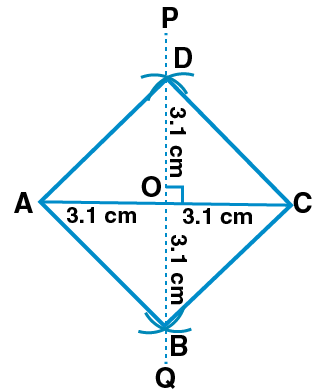# ML Aggarwal Solutions for Class 8 Maths Chapter 14: Constructions of Quadrilaterals

ML Aggarwal Solutions for Class 8 Maths Chapter 14 Constructions of Quadrilaterals mainly help students understand the steps to be followed in the construction of quadrilaterals. The steps of construction are explained in an elaborate manner to make it easy for the students to revise before the exams. To make it easier for the students, ML Aggarwal Solutions for Class 8 Maths Chapter 14 Constructions of Quadrilaterals PDF can be downloaded, with the help of links available below.

Chapter 14 has problems on constructing quadrilaterals based on the given dimensions. Students are advised to solve the textbook problems and refer to the solutions to cross check their answers. It helps students improve their problem solving and analytical thinking abilities, which are important from the exam perspective.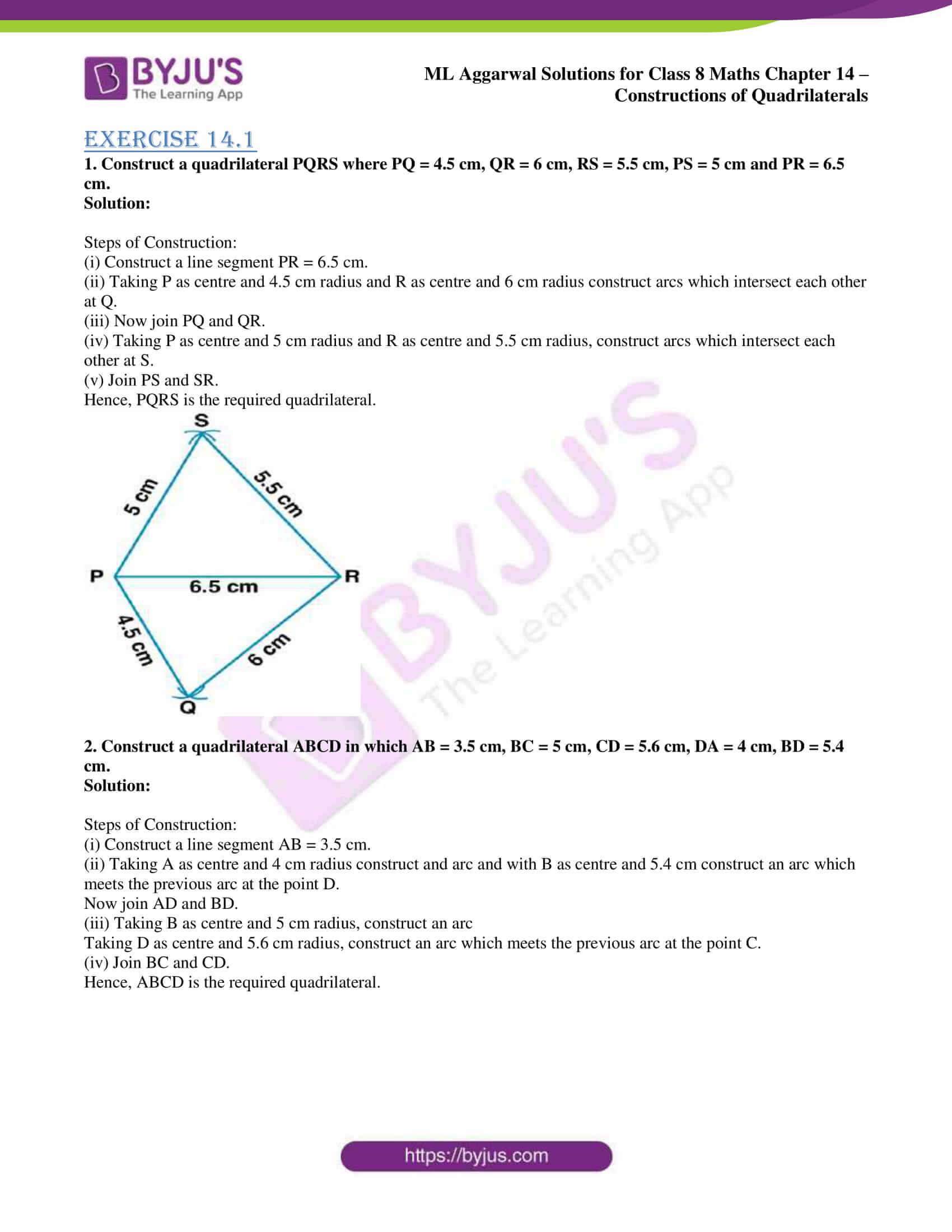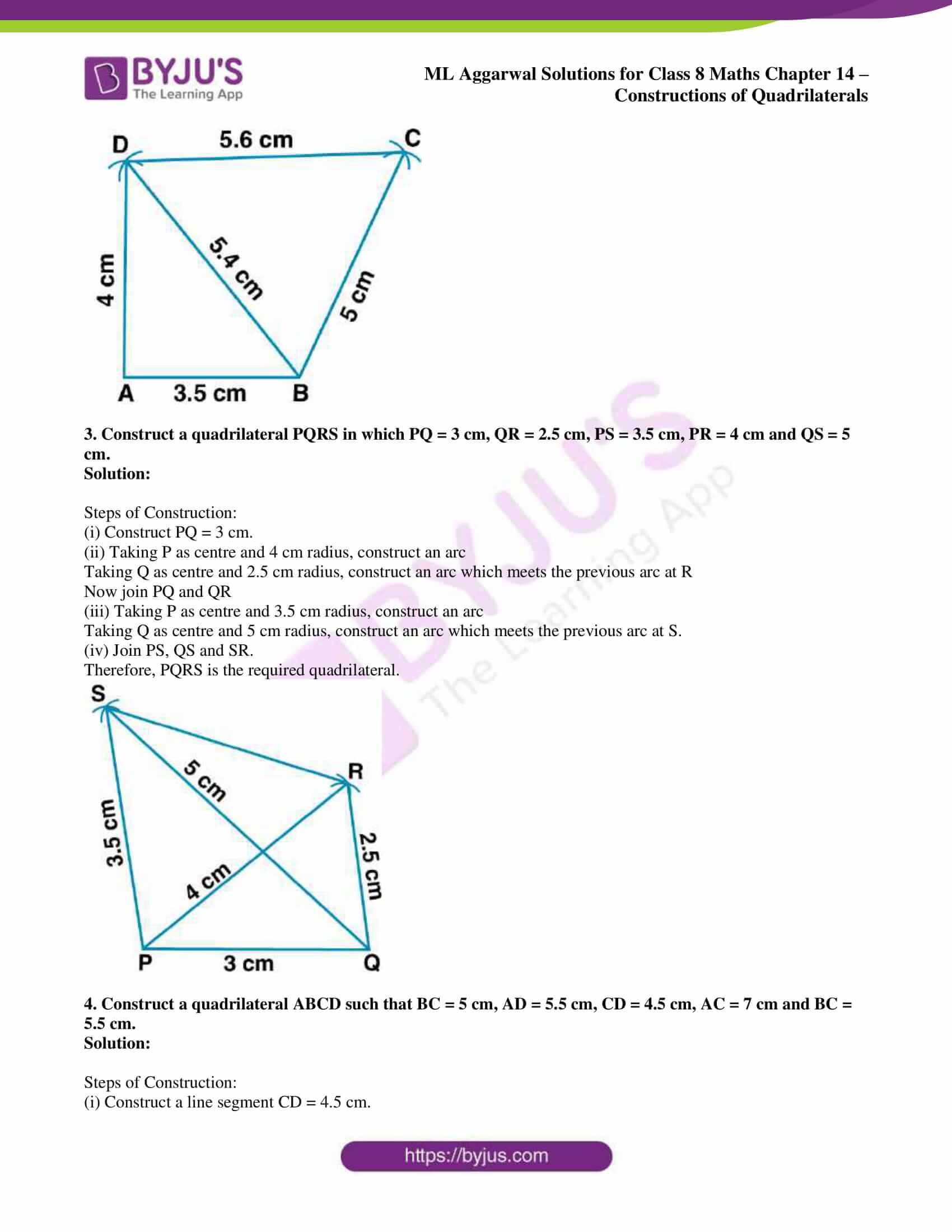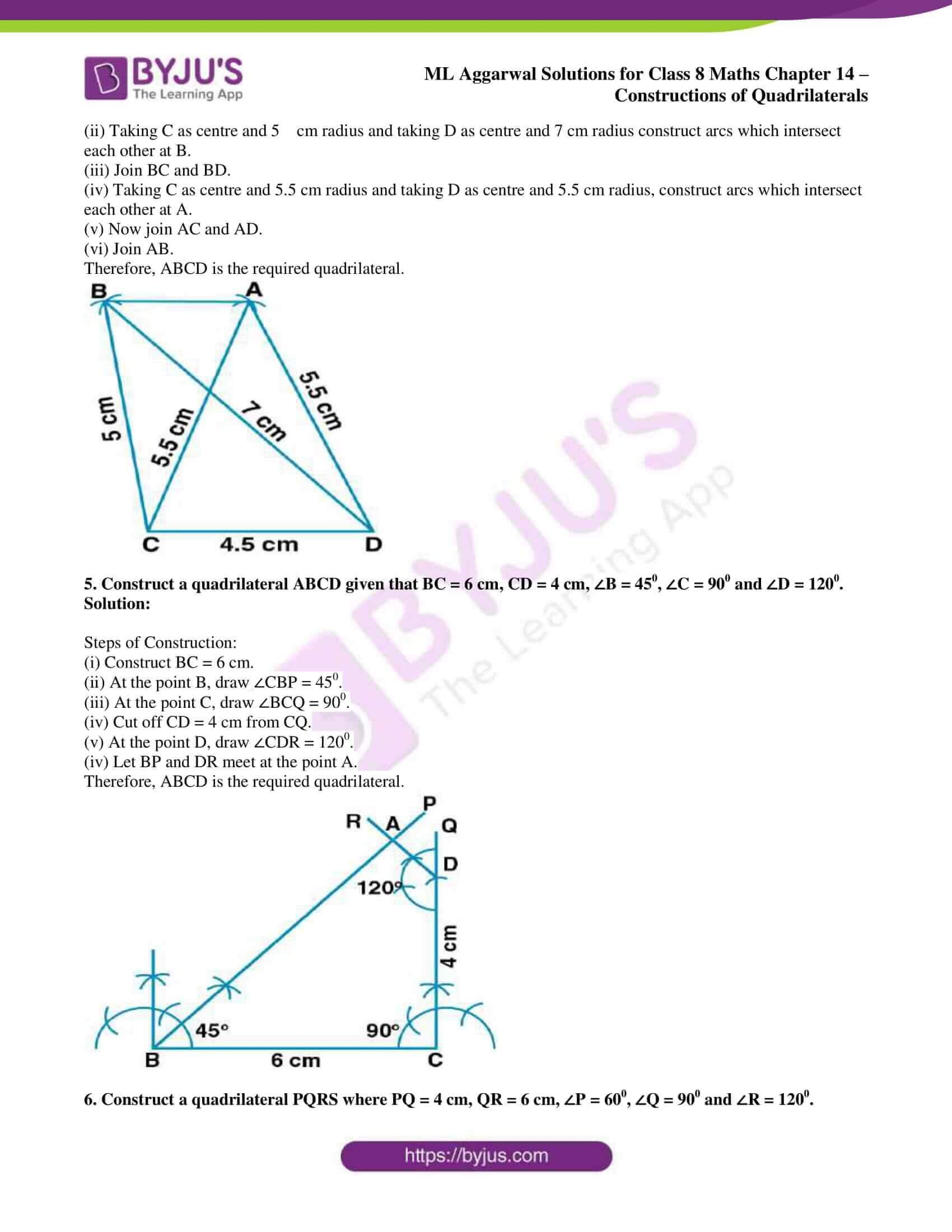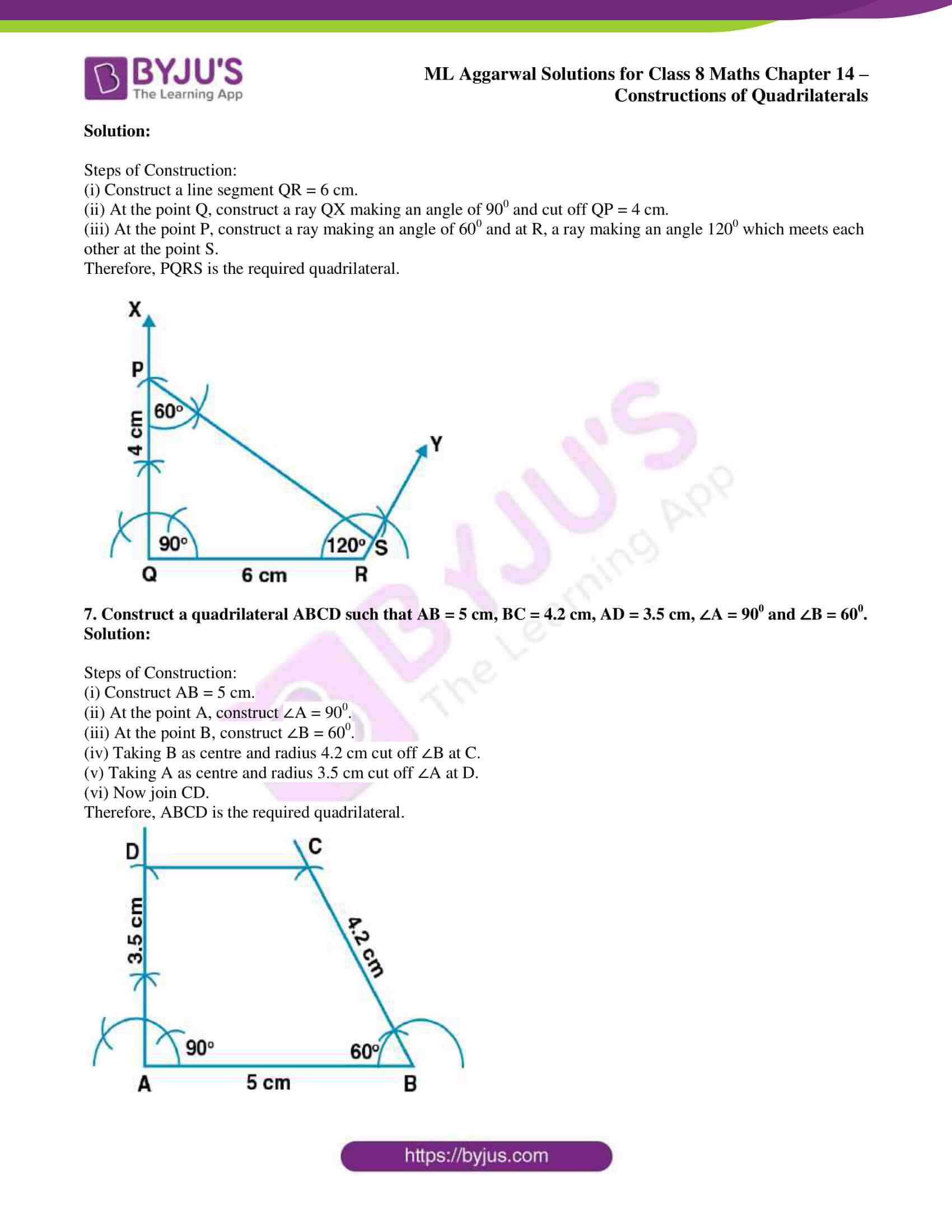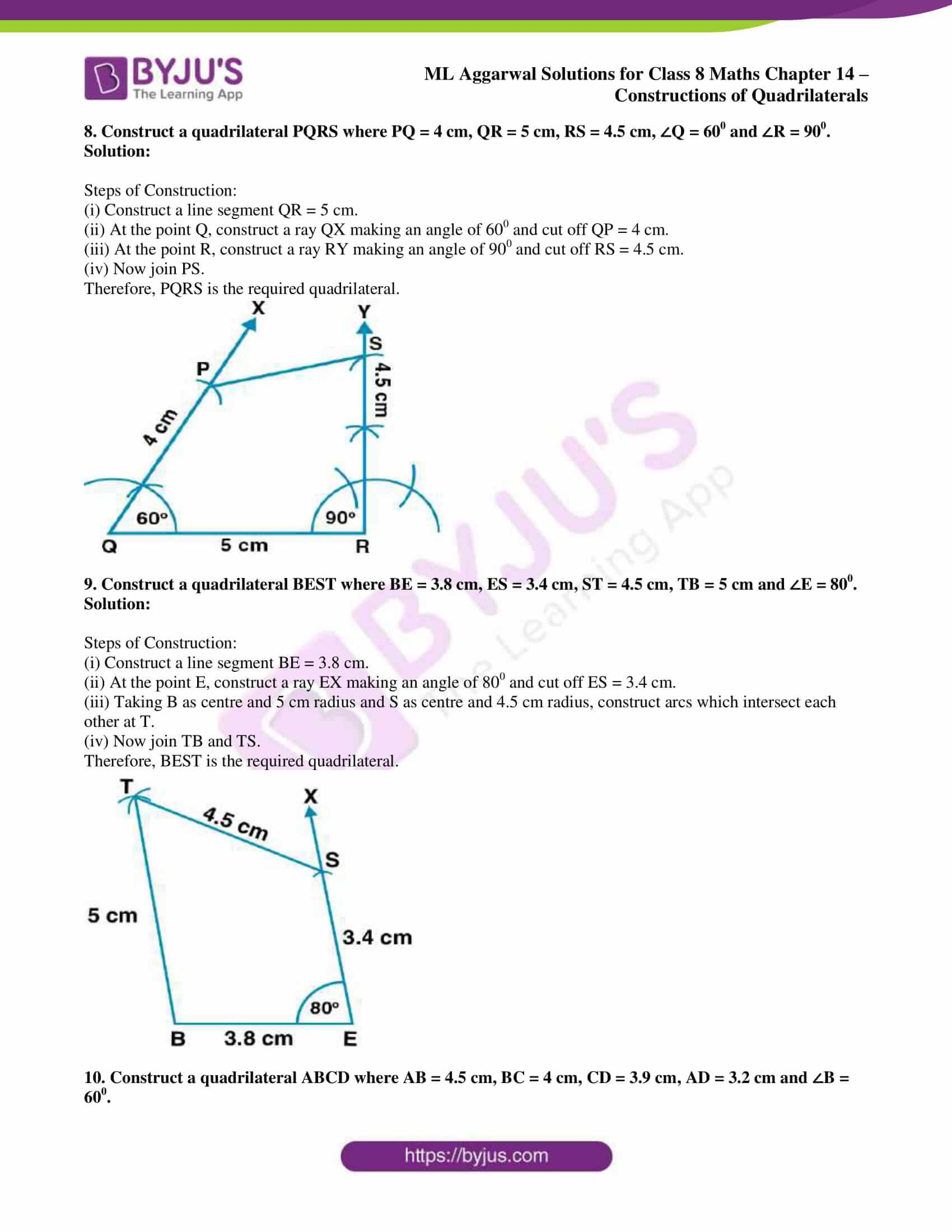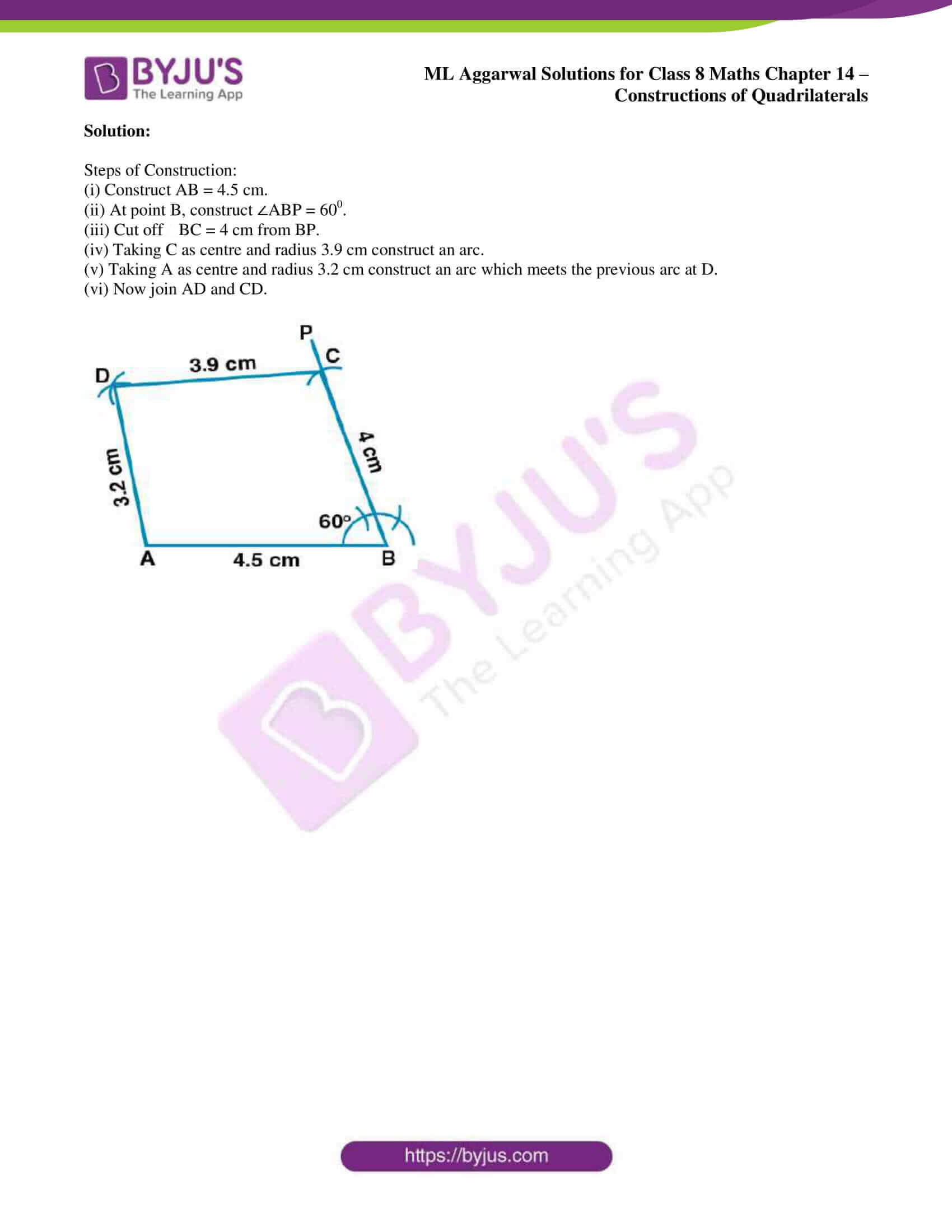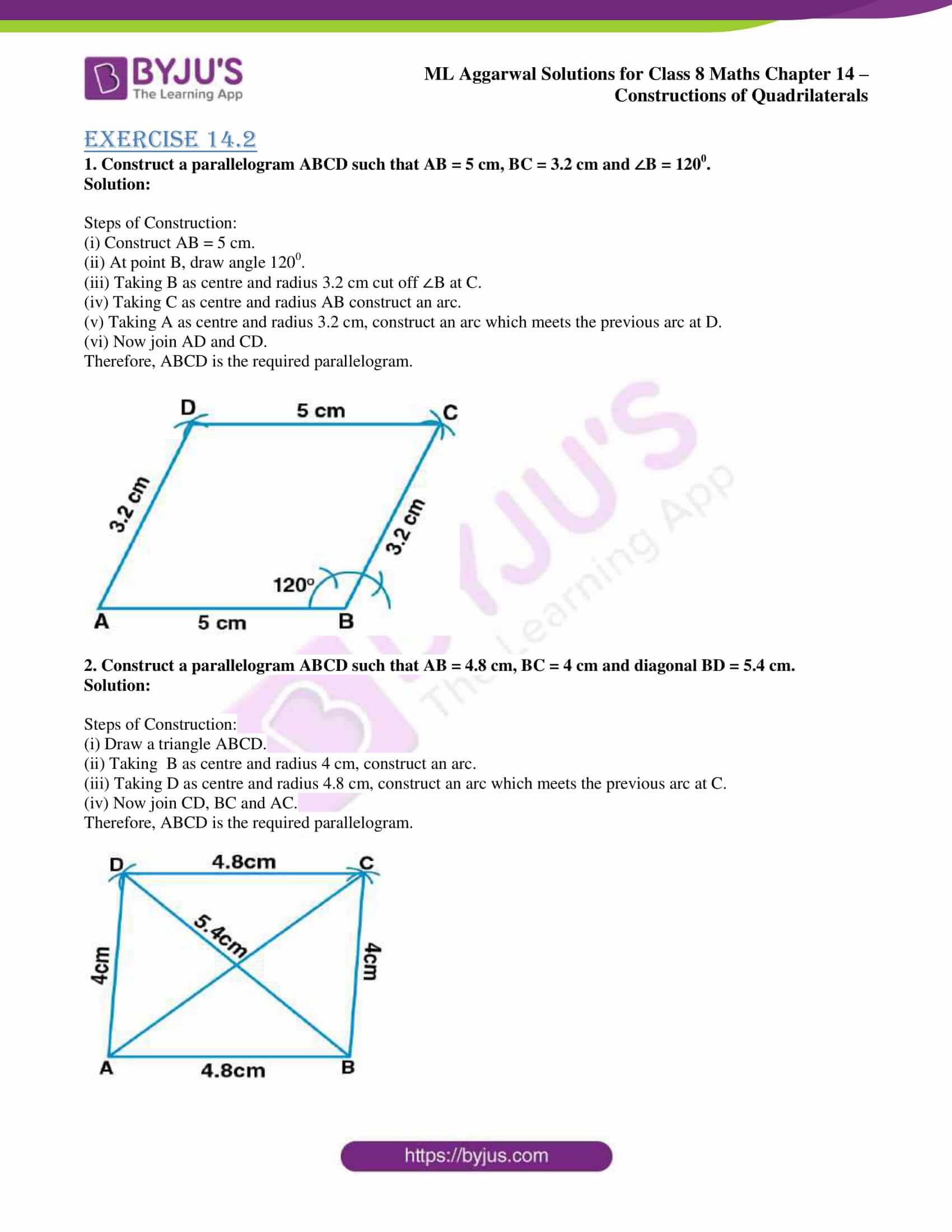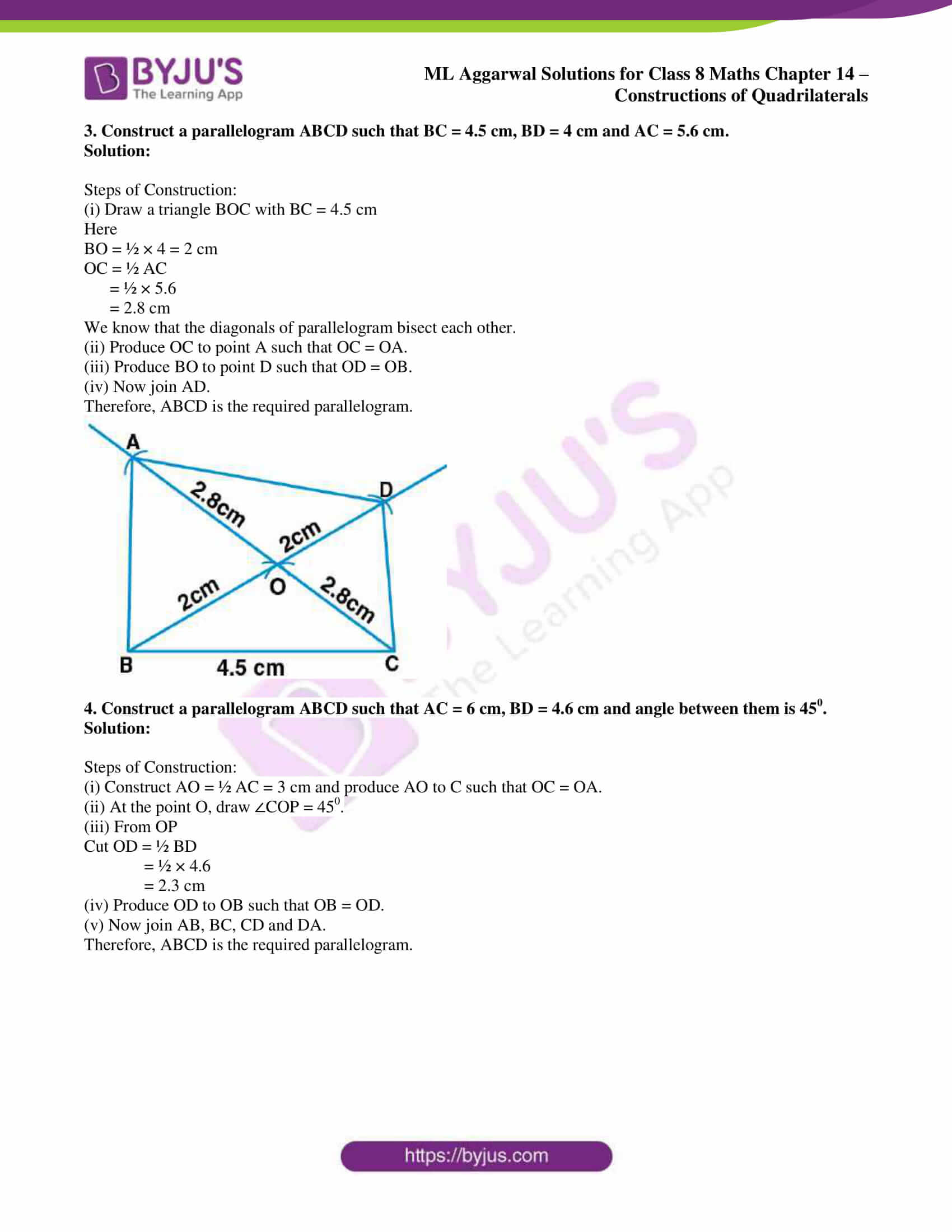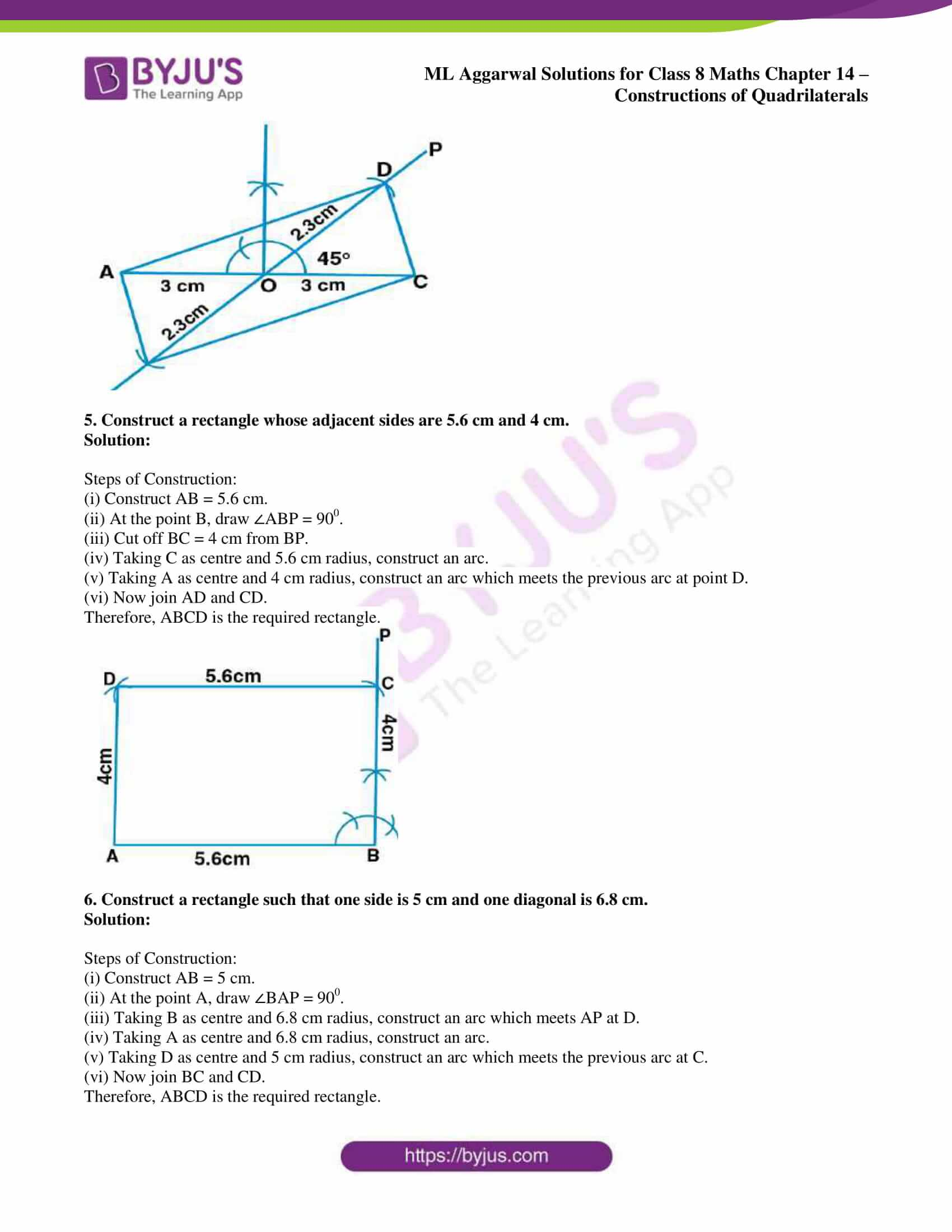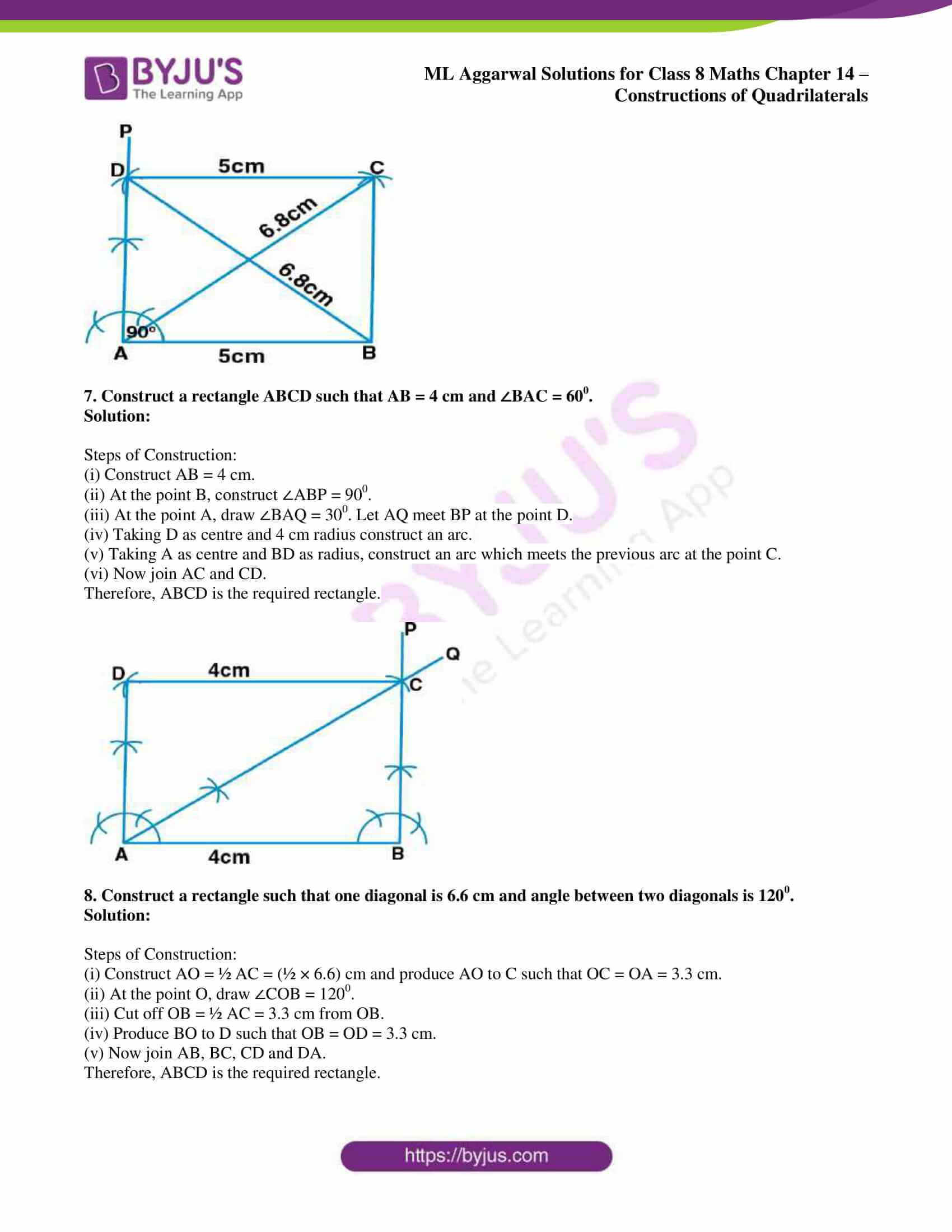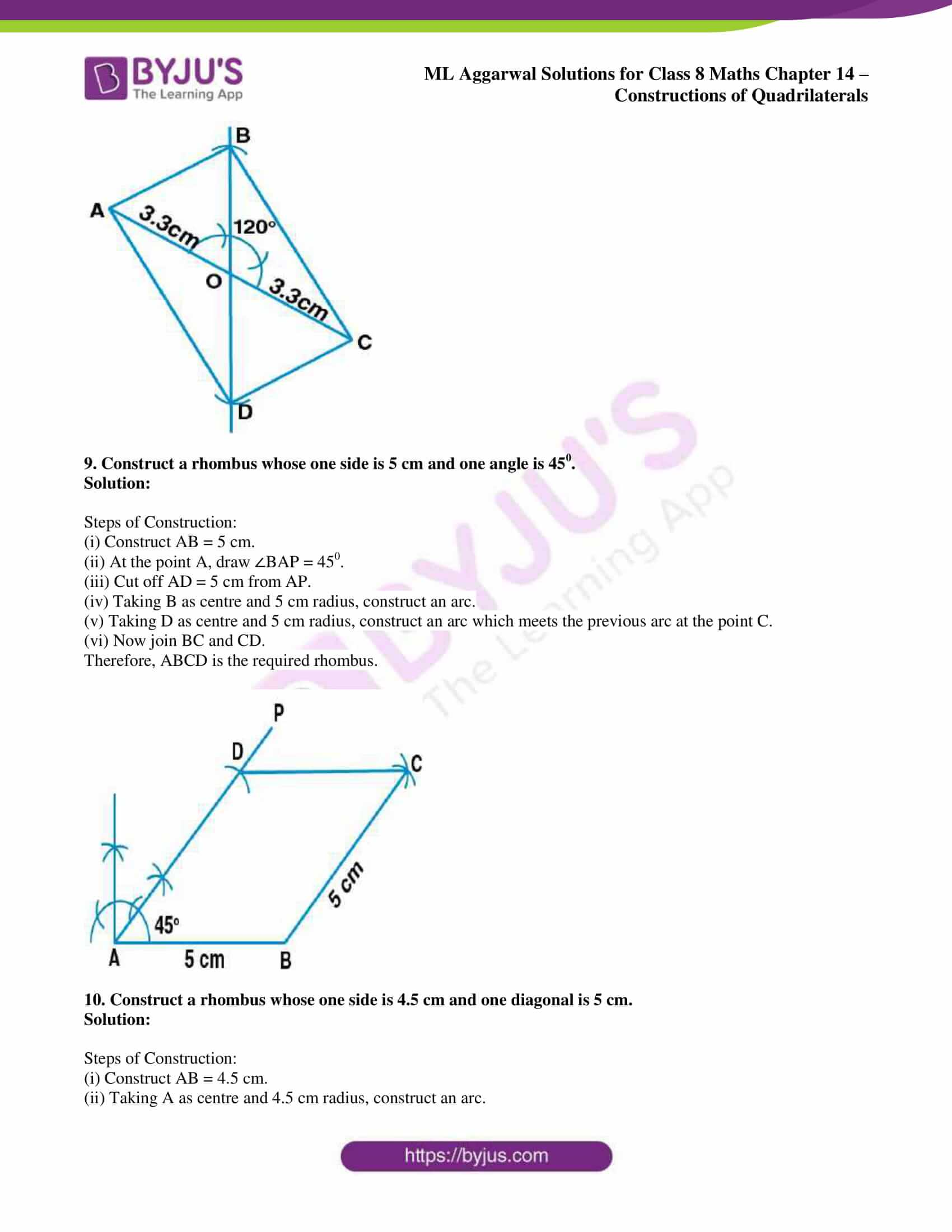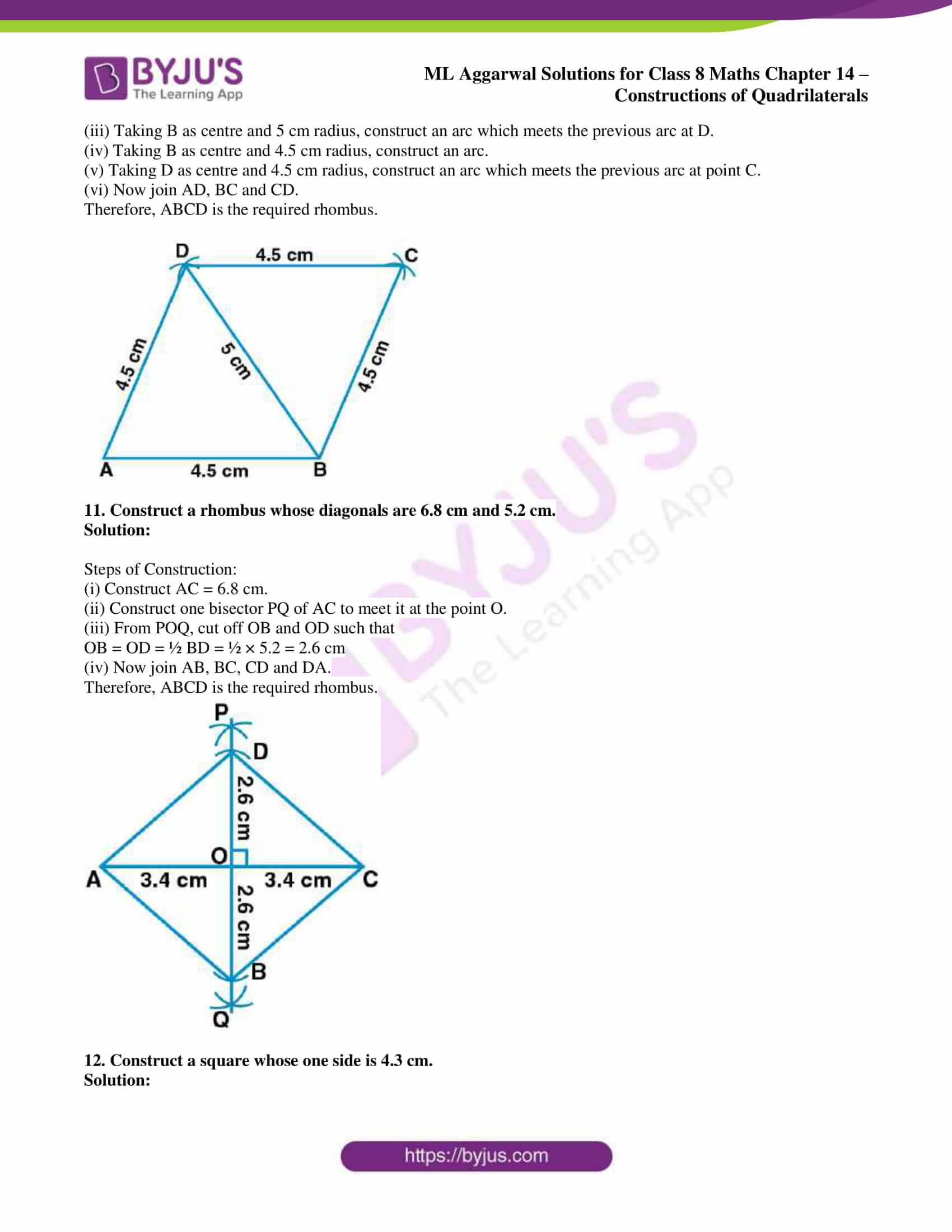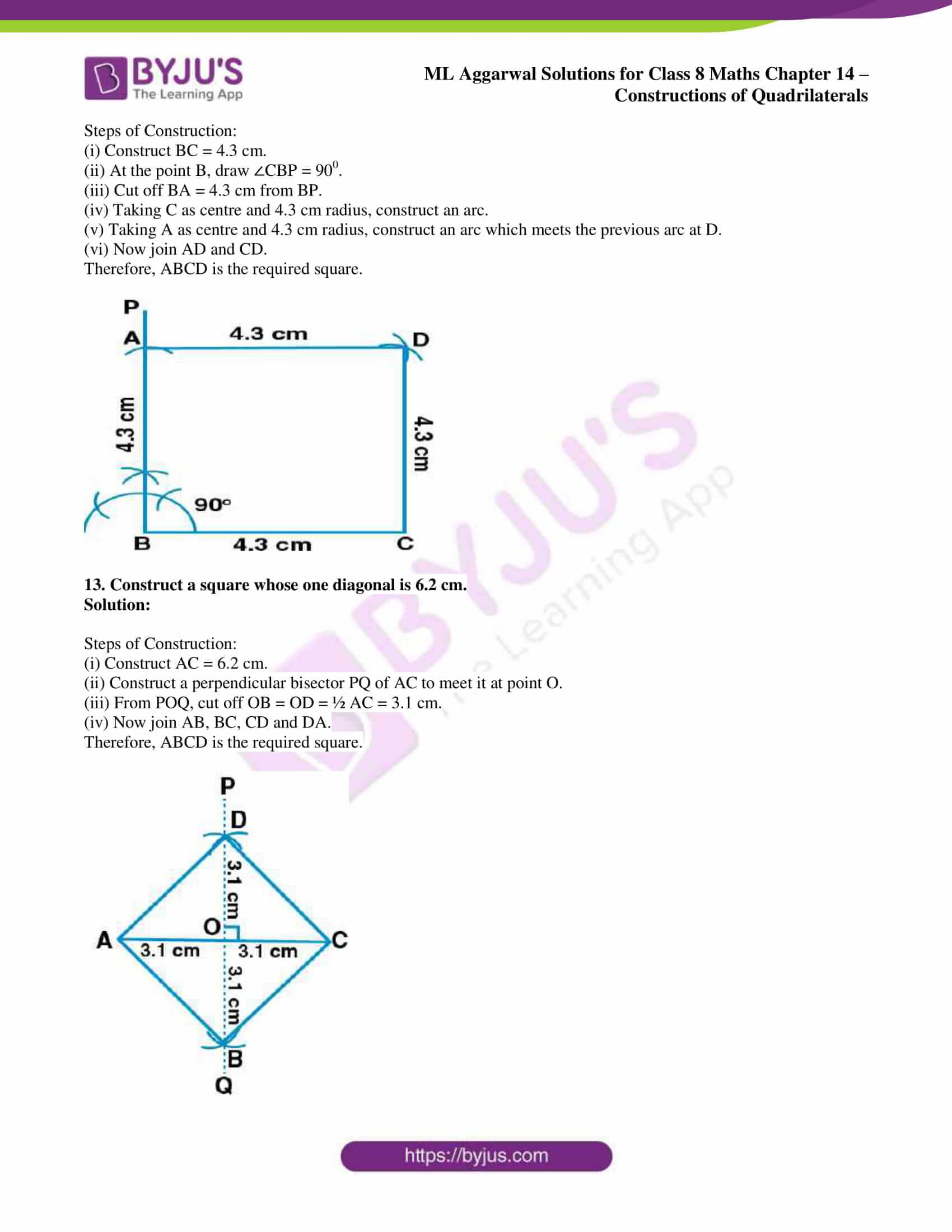## Access ML Aggarwal Solutions for Class 8 Maths Chapter 14: Constructions of Quadrilaterals

Exercise 14.1

1. Construct a quadrilateral PQRS where PQ = 4.5 cm, QR = 6 cm, RS = 5.5 cm, PS = 5 cm and PR = 6.5 cm.

Solution:

Steps of Construction:

(i) Construct a line segment PR = 6.5 cm.

(ii) Taking P as centre and 4.5 cm radius and R as centre and 6 cm radius construct arcs which intersect each other at Q.

(iii) Now join PQ and QR.

(iv) Taking P as centre and 5 cm radius and R as centre and 5.5 cm radius, construct arcs which intersect each other at S.

(v) Join PS and SR.

Hence, PQRS is the required quadrilateral.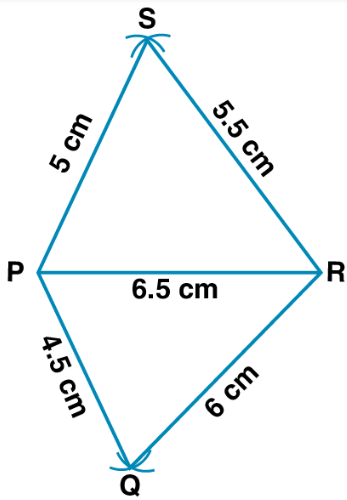2. Construct a quadrilateral ABCD in which AB = 3.5 cm, BC = 5 cm, CD = 5.6 cm, DA = 4 cm, BD = 5.4 cm.

Solution:

Steps of Construction:

(i) Construct a line segment AB = 3.5 cm.

(ii) Taking A as centre and 4 cm radius construct and arc and with B as centre and 5.4 cm construct an arc which meets the previous arc at the point D.

(iii) Taking B as centre and 5 cm radius, construct an arc

Taking D as centre and 5.6 cm radius, construct an arc which meets the previous arc at the point C.

(iv) Join BC and CD.

Hence, ABCD is the required quadrilateral.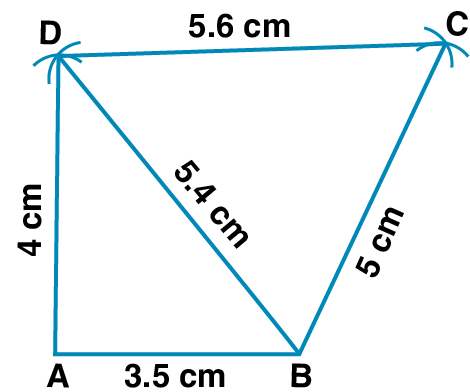3. Construct a quadrilateral PQRS in which PQ = 3 cm, QR = 2.5 cm, PS = 3.5 cm, PR = 4 cm and QS = 5 cm.

Solution:

Steps of Construction:

(i) Construct PQ = 3 cm.

(ii) Taking P as centre and 4 cm radius, construct an arc

Taking Q as centre and 2.5 cm radius, construct an arc which meets the previous arc at R

Now join PQ and QR

(iii) Taking P as centre and 3.5 cm radius, construct an arc

Taking Q as centre and 5 cm radius, construct an arc which meets the previous arc at S.

(iv) Join PS, QS and SR.

Therefore, PQRS is the required quadrilateral.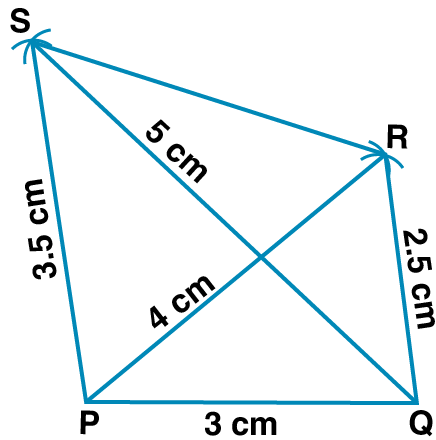4. Construct a quadrilateral ABCD such that BC = 5 cm, AD = 5.5 cm, CD = 4.5 cm, AC = 7 cm and BC = 5.5 cm.

Solution:

Steps of Construction:

(i) Construct a line segment CD = 4.5 cm.

(ii) Taking C as centre and 5 cm radius and taking D as centre and 7 cm radius construct arcs which intersect each other at B.

(iii) Join BC and BD.

(iv) Taking C as centre and 5.5 cm radius and taking D as centre and 5.5 cm radius, construct arcs which intersect each other at A.

(v) Now join AC and AD.

(vi) Join AB.

Therefore, ABCD is the required quadrilateral.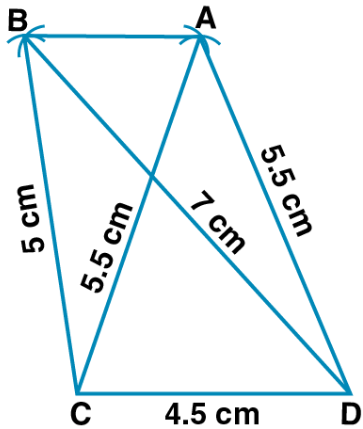5. Construct a quadrilateral ABCD given that BC = 6 cm, CD = 4 cm, ∠B = 450, ∠C = 900 and ∠D = 1200.

Solution:

Steps of Construction:

(i) Construct BC = 6 cm.

(ii) At the point B, draw ∠CBP = 450.

(iii) At the point C, draw ∠BCQ = 900.

(iv) Cut off CD = 4 cm from CQ.

(v) At the point D, draw ∠CDR = 1200.

(iv) Let BP and DR meet at the point A.

Therefore, ABCD is the required quadrilateral.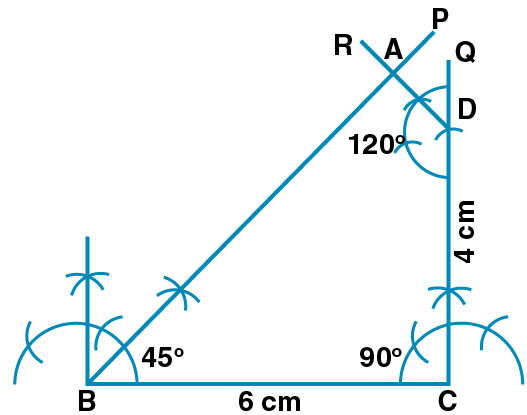6. Construct a quadrilateral PQRS where PQ = 4 cm, QR = 6 cm, ∠P = 600, ∠Q = 900 and ∠R = 1200.

Solution:

Steps of Construction:

(i) Construct a line segment QR = 6 cm.

(ii) At the point Q, construct a ray QX making an angle of 900 and cut off QP = 4 cm.

(iii) At the point P, construct a ray making an angle of 600 and at R, a ray making an angle 1200 which meets each other at the point S.

Therefore, PQRS is the required quadrilateral.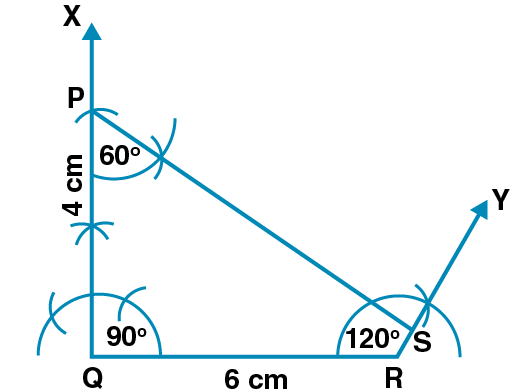7. Construct a quadrilateral ABCD such that AB = 5 cm, BC = 4.2 cm, AD = 3.5 cm, ∠A = 900 and ∠B = 600.

Solution:

Steps of Construction:

(i) Construct AB = 5 cm.

(ii) At the point A, construct ∠A = 900.

(iii) At the point B, construct ∠B = 600.

(iv) Taking B as centre and radius 4.2 cm cut off ∠B at C.

(v) Taking A as centre and radius 3.5 cm cut off ∠A at D.

(vi) Now join CD.

Therefore, ABCD is the required quadrilateral.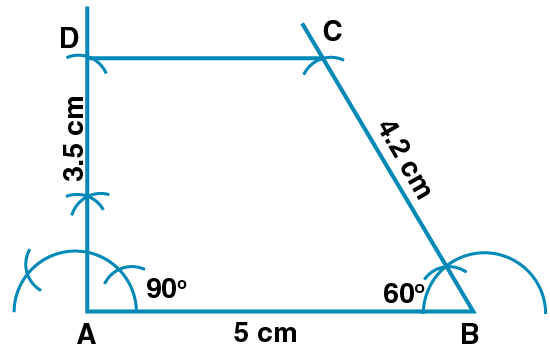8. Construct a quadrilateral PQRS where PQ = 4 cm, QR = 5 cm, RS = 4.5 cm, ∠Q = 600 and ∠R = 900.

Solution:

Steps of Construction:

(i) Construct a line segment QR = 5 cm.

(ii) At the point Q, construct a ray QX making an angle of 600 and cut off QP = 4 cm.

(iii) At the point R, construct a ray RY making an angle of 900 and cut off RS = 4.5 cm.

(iv) Now join PS.

Therefore, PQRS is the required quadrilateral.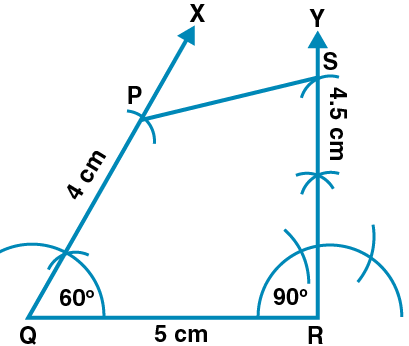9. Construct a quadrilateral BEST where BE = 3.8 cm, ES = 3.4 cm, ST = 4.5 cm, TB = 5 cm and ∠E = 800.

Solution:

Steps of Construction:

(i) Construct a line segment BE = 3.8 cm.

(ii) At the point E, construct a ray EX making an angle of 800 and cut off ES = 3.4 cm.

(iii) Taking B as centre and 5 cm radius and S as centre and 4.5 cm radius, construct arcs which intersect each other at T.

(iv) Now join TB and TS.

Therefore, BEST is the required quadrilateral.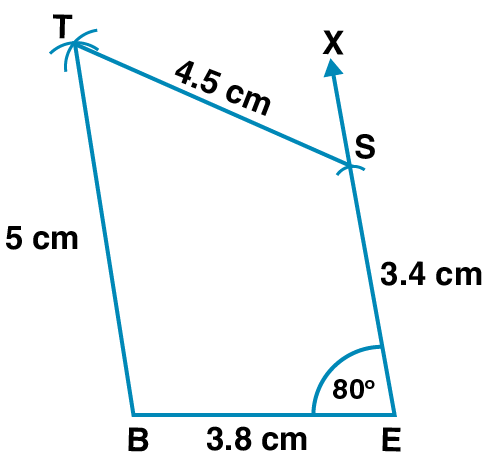10. Construct a quadrilateral ABCD where AB = 4.5 cm, BC = 4 cm, CD = 3.9 cm, AD = 3.2 cm and ∠B = 600.

Solution:

Steps of Construction:

(i) Construct AB = 4.5 cm.

(ii) At point B, construct ∠ABP = 600.

(iii) Cut off BC = 4 cm from BP.

(iv) Taking C as centre and radius 3.9 cm construct an arc.

(v) Taking A as centre and radius 3.2 cm construct an arc which meets the previous arc at D.

(vi) Now join AD and CD.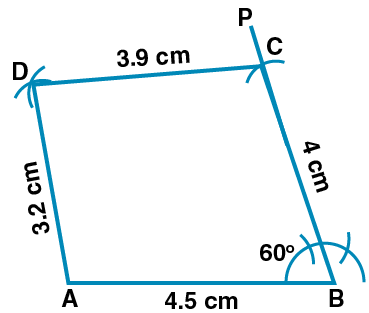Exercise 14.2

1. Construct a parallelogram ABCD such that AB = 5 cm, BC = 3.2 cm and ∠B = 1200.

Solution:

Steps of Construction:

(i) Construct AB = 5 cm.

(ii) At point B, draw angle 1200.

(iii) Taking B as centre and radius 3.2 cm cut off ∠B at C.

(iv) Taking C as centre and radius AB construct an arc.

(v) Taking A as centre and radius 3.2 cm, construct an arc which meets the previous arc at D.

(vi) Now join AD and CD.

Therefore, ABCD is the required parallelogram.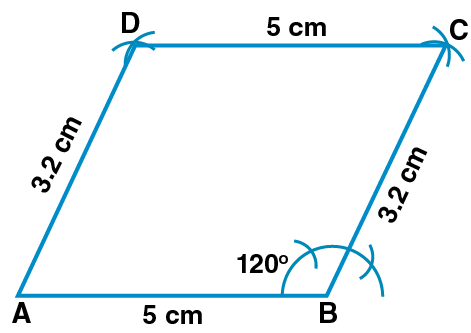2. Construct a parallelogram ABCD such that AB = 4.8 cm, BC = 4 cm and diagonal BD = 5.4 cm.

Solution:

Steps of Construction:

(i) Draw a triangle ABCD.

(ii) Taking B as centre and radius 4 cm, construct an arc.

(iii) Taking D as centre and radius 4.8 cm, construct an arc which meets the previous arc at C.

(iv) Now join CD, BC and AC.

Therefore, ABCD is the required parallelogram.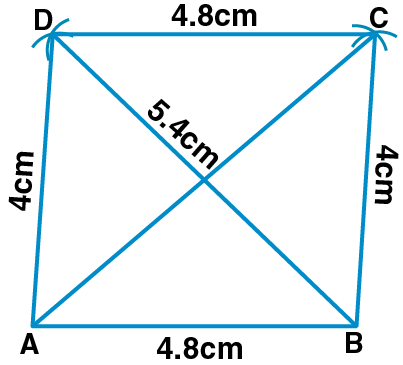3. Construct a parallelogram ABCD such that BC = 4.5 cm, BD = 4 cm and AC = 5.6 cm.

Solution:

Steps of Construction:

(i) Draw a triangle BOC with BC = 4.5 cm

Here

BO = ½ × 4 = 2 cm

OC = ½ AC

= ½ × 5.6

= 2.8 cm

We know that the diagonals of parallelogram bisect each other.

(ii) Produce OC to point A such that OC = OA.

(iii) Produce BO to point D such that OD = OB.

Therefore, ABCD is the required parallelogram.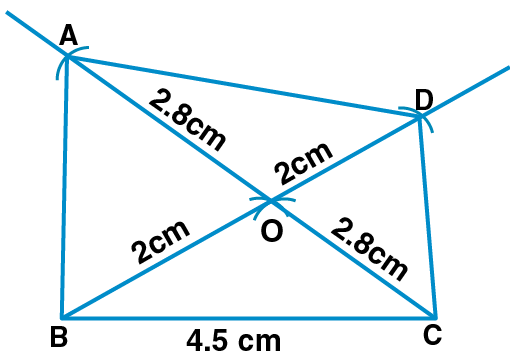4. Construct a parallelogram ABCD such that AC = 6 cm, BD = 4.6 cm and angle between them is 450.

Solution:

Steps of Construction:

(i) Construct AO = ½ AC = 3 cm and produce AO to C such that OC = OA.

(ii) At the point O, draw ∠COP = 450.

(iii) From OP

Cut OD = ½ BD

= ½ × 4.6

= 2.3 cm

(iv) Produce OD to OB such that OB = OD.

(v) Now join AB, BC, CD and DA.

Therefore, ABCD is the required parallelogram.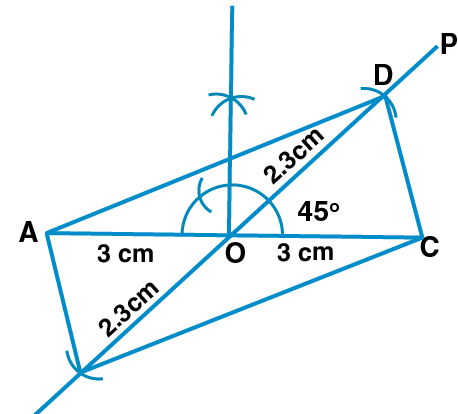5. Construct a rectangle whose adjacent sides are 5.6 cm and 4 cm.

Solution:

Steps of Construction:

(i) Construct AB = 5.6 cm.

(ii) At the point B, draw ∠ABP = 900.

(iii) Cut off BC = 4 cm from BP.

(iv) Taking C as centre and 5.6 cm radius, construct an arc.

(v) Taking A as centre and 4 cm radius, construct an arc which meets the previous arc at point D.

(vi) Now join AD and CD.

Therefore, ABCD is the required rectangle.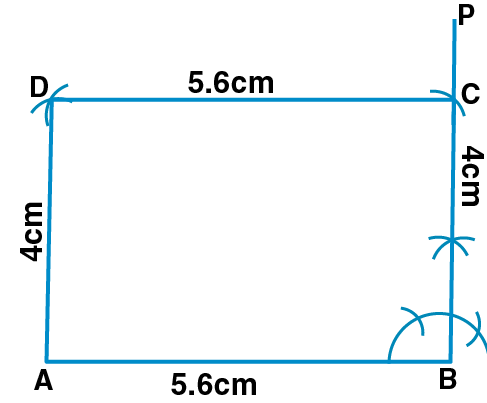6. Construct a rectangle such that one side is 5 cm and one diagonal is 6.8 cm.

Solution:

Steps of Construction:

(i) Construct AB = 5 cm.

(ii) At the point A, draw ∠BAP = 900.

(iii) Taking B as centre and 6.8 cm radius, construct an arc which meets AP at D.

(iv) Taking A as centre and 6.8 cm radius, construct an arc.

(v) Taking D as centre and 5 cm radius, construct an arc which meets the previous arc at C.

(vi) Now join BC and CD.

Therefore, ABCD is the required rectangle.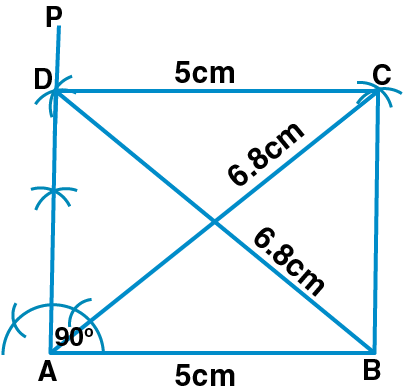7. Construct a rectangle ABCD such that AB = 4 cm and ∠BAC = 600.

Solution:

Steps of Construction:

(i) Construct AB = 4 cm.

(ii) At the point B, construct ∠ABP = 900.

(iii) At the point A, draw ∠BAQ = 300. Let AQ meet BP at the point D.

(iv) Taking D as centre and 4 cm radius construct an arc.

(v) Taking A as centre and BD as radius, construct an arc which meets the previous arc at the point C.

(vi) Now join AC and CD.

Therefore, ABCD is the required rectangle.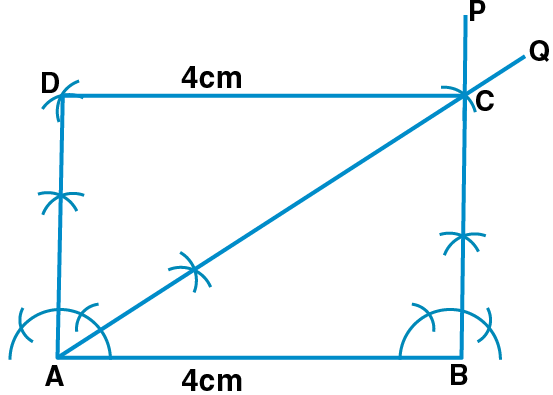8. Construct a rectangle such that one diagonal is 6.6 cm and angle between two diagonals is 1200.

Solution:

Steps of Construction:

(i) Construct AO = ½ AC = (½ × 6.6) cm and produce AO to C such that OC = OA = 3.3 cm.

(ii) At the point O, draw ∠COB = 1200.

(iii) Cut off OB = ½ AC = 3.3 cm from OB.

(iv) Produce BO to D such that OB = OD = 3.3 cm.

(v) Now join AB, BC, CD and DA.

Therefore, ABCD is the required rectangle.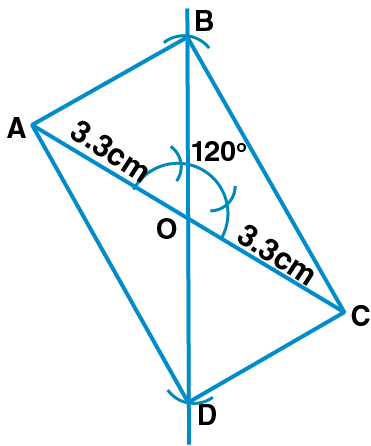9. Construct a rhombus whose one side is 5 cm and one angle is 450.

Solution:

Steps of Construction:

(i) Construct AB = 5 cm.

(ii) At the point A, draw ∠BAP = 450.

(iii) Cut off AD = 5 cm from AP.

(iv) Taking B as centre and 5 cm radius, construct an arc.

(v) Taking D as centre and 5 cm radius, construct an arc which meets the previous arc at the point C.

(vi) Now join BC and CD.

Therefore, ABCD is the required rhombus.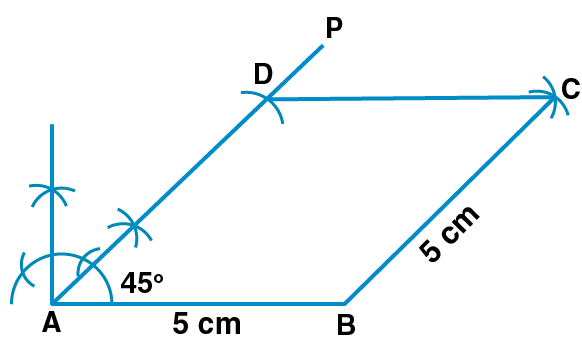10. Construct a rhombus whose one side is 4.5 cm and one diagonal is 5 cm.

Solution:

Steps of Construction:

(i) Construct AB = 4.5 cm.

(ii) Taking A as centre and 4.5 cm radius, construct an arc.

(iii) Taking B as centre and 5 cm radius, construct an arc which meets the previous arc at D.

(iv) Taking B as centre and 4.5 cm radius, construct an arc.

(v) Taking D as centre and 4.5 cm radius, construct an arc which meets the previous arc at point C.

(vi) Now join AD, BC and CD.

Therefore, ABCD is the required rhombus.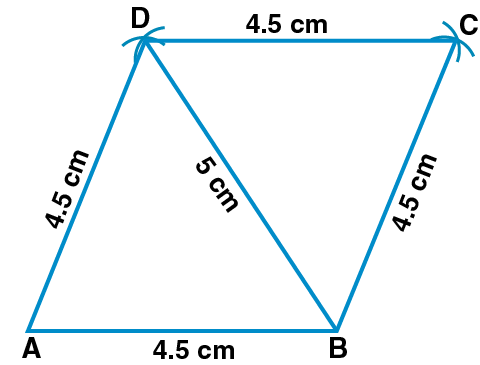11. Construct a rhombus whose diagonals are 6.8 cm and 5.2 cm.

Solution:

Steps of Construction:

(i) Construct AC = 6.8 cm.

(ii) Construct one bisector PQ of AC to meet it at the point O.

(iii) From POQ, cut off OB and OD such that

OB = OD = ½ BD = ½ × 5.2 = 2.6 cm

(iv) Now join AB, BC, CD and DA.

Therefore, ABCD is the required rhombus.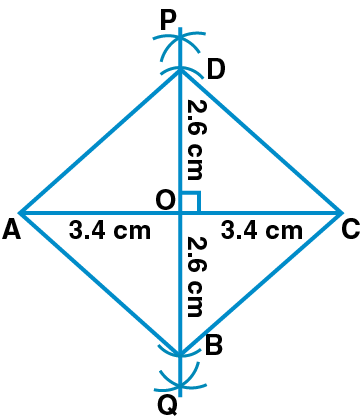12. Construct a square whose one side is 4.3 cm.

Solution:

Steps of Construction:

(i) Construct BC = 4.3 cm.

(ii) At the point B, draw ∠CBP = 900.

(iii) Cut off BA = 4.3 cm from BP.

(iv) Taking C as centre and 4.3 cm radius, construct an arc.

(v) Taking A as centre and 4.3 cm radius, construct an arc which meets the previous arc at D.

(vi) Now join AD and CD.

Therefore, ABCD is the required square.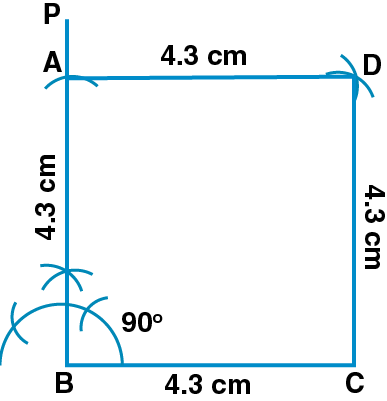13. Construct a square whose one diagonal is 6.2 cm.

Solution:

Steps of Construction:

(i) Construct AC = 6.2 cm.

(ii) Construct a perpendicular bisector PQ of AC to meet it at point O.

(iii) From POQ, cut off OB = OD = ½ AC = 3.1 cm.

(iv) Now join AB, BC, CD and DA.

Therefore, ABCD is the required square.